Courses

# What is Percentage Composition ? Class 11 Notes | EduRev

## Class 11 : What is Percentage Composition ? Class 11 Notes | EduRev

The document What is Percentage Composition ? Class 11 Notes | EduRev is a part of the Class 11 Course Chemistry Class 11.
All you need of Class 11 at this link: Class 11

What is Percentage Composition?

• The percentage composition of a given compound is nothing but the ratio of the amount of each element to the total amount of individual elements present in the compound.
• We then multiply this value by 100. Here, we measure the quantity in terms of grams of the elements present in the solution.
• The per cent composition of any compound is an expression of its composition in terms of all the elements present. The significance of this composition calculation is found in the chemical analysis.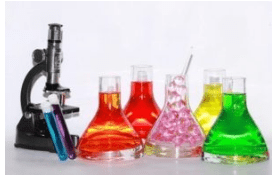Different Compounds

Formula for Percentage Composition
• We can express the percentage composition of a given element using the formula below:
% CE = gE/g× 100
• Here, % CE is the percentage composition of element E. This is the value that we are going to calculate. The numerator on the right side indicates the total amount of element E present in the compound. On the other hand, the denominator is an expression for the total amount of all the elements present in the compound.
• We multiply this ratio by 100 to get the percentage form of the composition. Let us now look at the mass percentage of composition in more details. We will also look at its importance.
Mass Percentage Composition
• We use the concept of mass percentage composition to denote the concentration of an element in a compound or a component in a mixture. We use this term to signify the total per cent by mass of each element that is present in a compound.
• It is important to note that we can calculate the mass percentage composition by dividing the mass of a component by the total mass of the mixture. This ratio is then to be multiplied by 100. We also call it the mass per cent (w/w) %.
Importance of Mass Percentage
• For many scientific and practical reasons, we ought to know what quantity of an element is present in a given compound. This is indispensable for chemists to get to the empirical formula of many compounds.
• This formula helps in showing the lowest whole number of moles and the relative number of atoms of each element in a compound. With the help of the empirical formula, chemists can also calculate the actual molecular formula. This formula shows the exact number of atoms in the compound.
Determining the Mass Percent from a Chemical Formula
• We will explain this section with the help of an example. It will clear your fundamentals on how to calculate the mass per cent.
Let us consider glucose. The formula for glucose is C6H12O6.
Let us calculate the mass per cent of each of the elements in glucose.
• From the formula, we can see the number of moles of each of the elements. Glucose has 6 carbon atoms, 12 hydrogen atoms and also 6 oxygen atoms.
Let us now multiply each of the atoms by its molar mass. Thus, we arrive at the mass of each of the elements in glucose.
• We now divide each mass by mass of 1 mole of glucose to arrive at the mass fraction of each element. Multiplying this value by 100 gives the mass percentage of the elements.
Example
• Each mole of carbon has a mass of 12.01 g/mol of carbon.
This, we know, from the periodic table. So, 6 moles of carbon will have 12.01 g/mol × 6 = 72.06 g of Carbon.
Similarly, 1 mole of Hydrogen has a mass of 1.008 g/mol of Hydrogen. Therefore, 12 moles of Hydrogen will have the mass of 12 × 1.008 = 12.096 g of Hydrogen.
• Going by the same logic for Oxygen, 1 mole of oxygen has a mass of 16.00 g/mol.
Therefore, 6 moles of oxygen will have 16.00 × 6 = 96 g of Oxygen
Thus, 1 mole of Glucose (C6H12O6) has a total mass of 72.06 + 12.096 + 96 = 180.16 g/mol
Calculating the Mass Percentage
• To find out the mass % of the three elements of glucose, let us first calculate the mass fraction of each element in 1 mole of glucose. So, what do we mean by the mass fraction? It is the mass that each element contributes to the total mass of glucose. By multiplying this by 100, we get the mass percentage of each element.
Mass fraction of Carbon = 72.06 g/180.16 g = 0.4000
Therefore, mass % of Carbon= 0.4000 × 100 = 40.00%
Mass fraction of Hydrogen = 12.096/180.16 = 0.06714
Therefore, mass % of Hydrogen= 0.06714 × 100 = 6.714%
Mass fraction of Oxygen =96/180.16 = 0.53286
Therefore, mass% of Oxygen = 0.53286 × 100 = 53.286%
• It is interesting to note that even if carbon and oxygen have an equal number of moles of the compound, their mass percentages are different. Oxygen has a higher value in this as its molar mass is higher than that of carbon.

Solved Example

Q. Find the percent composition of each element in water.
Solution. We know that the chemical formula for water is H2O. Let us now calculate the molar mass of water. The molar mass of Oxygen = 16.00 × 1 = 16 g/mole and of Hydrogen = 1.01 × 2 = 2.02 g/mole.
Now, using the molar mass of each of the given elements, we find out the percentage composition of each element in H2O. It is given as the ratio of the grams of the element to the grams of the total element in the compound, multiplied by 100.
Calculating the percentage composition of Hydrogen:
% H = 2.02/18.02 × 100
Therefore, % H= 11.21 %
Calculating the percentage composition of Oxygen:
Therefore, % O = 16/18.02 × 100 = 88.79 %

Try yourself:The percentage of oxygen in is NaOH

Try yourself:The percentage of nitrogen in urea is about [KCET 2001]

Empirical Formulas
• The Empirical formulas express the simplest atomic ratio between the elements in the compound. Such formulas are called empirical formulas.
• An empirical formula does not necessarily represent the actual numbers of atoms present in a molecule of a compound it represents only the ratio between those numbers.
• So it may or may not represent the actual formula of the molecule. It can be deduced by knowing the weight % of all the constituent elements with their atomic masses for the given compound.
Example: C6H12O6, CH3COOH, HCHO
All have the same empirical formula CH2O, but they are different.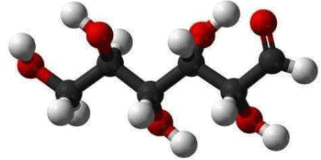Structure of Glucose

The empirical formula of a compound can be determined by the following steps:
(i) Write the name of detected elements in the column 1 present in the compound. Write the corresponding atomic mass in column 2.
(ii) Write the experimentally determined percentage composition by weight of each element present in the compound in column 3.
(iii) Divide the percentage of each element by its atomic weight to get the relative number of atoms of each element in column 4.
(iv) Divide each number obtained for the respective elements in step (iii) by the smallest number among those numbers so as to get the simplest ratio in column 5.
(v) If any number obtained in step (iv) is not a whole number then multiply all the numbers by a suitable integer to get the whole-number ratio. This ratio will be the simplest ratio of the atoms of different elements present in the compound. The empirical formula of the compound can be written with the help of this ratio in column-6.

Example 1. A compound contains C = 71.23%, H = 12.95% and O = 15.81%. What is the empirical formula of the compound?
Solution.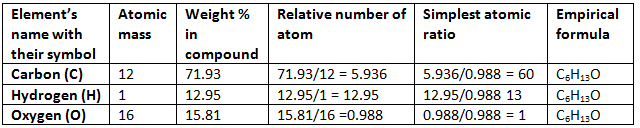Example 2. The simplest formula of a compound containing 50% of element X (Atomic mass = 10) and 50% of the element Y (Atomic mass = 20) is:
(A) XY
(B) X2Y
(C) XY2
(D) X2Y3
Solution.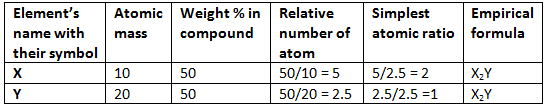Hence (B) is correct.

Molecular Formulas
• The actual numbers of atoms of each element that occur in the smallest freely existing unit or molecule of the compound are expressed by the molecular formula of the compound.
• The molecular formula of a compound may be the empirical formula, or it may be a multiple of the empirical formula
• Conclusively, the formula which represents the actual number of each individual atom in any molecule is known as the molecular formula.
• Molecular formula = (Empirical formula)n
Molecular weight = Empirical formula weight × n
• If the vapour density of the substance is known, its molecular weight can be calculated by using the equation:
2 × Vapour density = Molecular weight
• Example: The molecular formula of butene, C4H8, shows that each freely existing molecule of butene contains four atoms of carbon and eight atoms of hydrogen. Its empirical formula is CH2.
One molecule of ethylene (molecular formula C2H4) contains two atoms of carbon and four atoms of hydrogen. Its empirical formula is CH2. Both have the same empirical formula, yet they are different compounds with different molecular formulas.
Butene is C4H8 or four times the empirical formula, ethylene is C2H4 or twice the empirical formula.
• For certain compounds, the molecular formula and the empirical formula may be the same.
Example: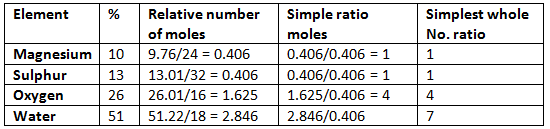Hence the empirical formula is MgSO4.7H2O.

For Writing Molecular Formula

• Determine empirical formula mass Add the atomic masses of various atoms present in the empirical formula.
• Divide Molar mass by empirical formula mass which will give the ‘n’ factor.
• Molecular formula = n × Empirical formula

Example 3. A compound contains 4.07 % hydrogen, 24.27 % carbon and 71.65 % chlorine. Its molar mass is 98.96 g. What are its empirical and molecular formulas?
Solution.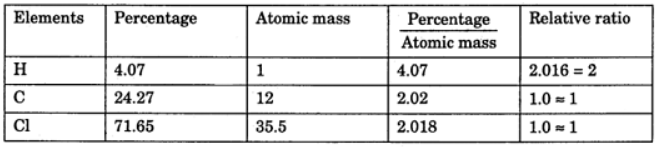Empirical formula = CH2Cl
Molecular formula = n * Empirical formula
n = 98.96 / 48.5 = 2.04 = 2 (approx)
Molecular formula = 2 * CH2Cl = C2H4Cl2

Example 4. A compound of carbon, hydrogen and nitrogen contains these elements in the ratio 9:1:3.5. Calculate the empirical formula. If its molecular mass is 108, what is the molecular formula?
Solution.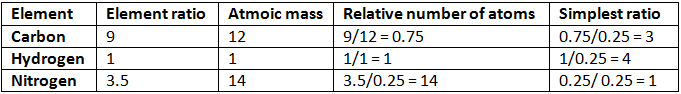Empirical formula = C3H4N
Empirical formula mass = (3 × 12) + (4 × 1) + 14 = 54
n = mol. mass/Emp. mass
= 108/54 = 2
Thus, molecular formula of the compound = 2 × Empirical formula
= 2 × C3H4N
= C6H8N2

Example 5. 2.38 gm of uranium was heated strongly in a current of air. The resulting oxide weighed 2.806 g. Determine the empirical formula of the oxide. (At. mass U = 238; O = 16).
Solution.
Step 1: To calculate the percentage of uranium and oxygen in the oxide.
2.806 g of the oxide contain uranium = 2.38 g
∴ Percentage of uranium = 2.38/2.806 × 100 = 84.82
Hence, the percentage of oxygen in the oxide
= 100.00 – 84.82 = 15.18
Step 2: To calculate the empirical formula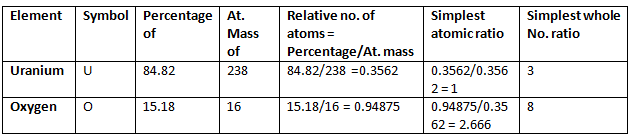Hence, the empirical formula of the oxide is U3O8.

Example 6. Chemical analysis of a carbon compound gave the following percentage composition by weight of the elements present. Carbon 10.06%, hydrogen 0.84%, chlorine 89.10%. Calculate the empirical formula of the compound.
Solution.

Step 1: Percentage of the elements present.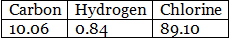Step 2: Dividing the percentage compositions by the respective atomic weights of the elements.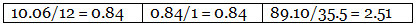Step 3: Dividing each value in step 2 by the smallest number among them to get simple atomic ratio.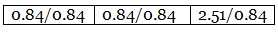Step 4: Ratio of the atoms present in the molecule.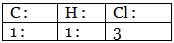∴ The empirical formula of the compound C1H1Cl3 or CHCl3.

Example 7. A carbon compound on analysis gave the following percentage composition. Carbon 14.5%, hydrogen 1.8%, chlorine 64.46%, oxygen 19.24%. Calculate the empirical formula of the compound.
Solution.
Step 1: Percentage composition of the elements present in the compound.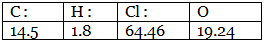Step 2: Dividing by the respective atomic weights.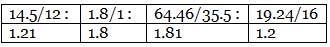Step 3: Dividing the values in step 2 among them by the smallest number.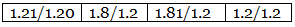Step 4: Multiplication by a suitable integer to get the whole-number ratio.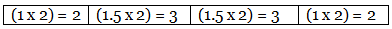∴ The simplest ratio of the atoms of different elements in the compound.
C : H : Cl : O = 2 : 3 : 3 : 2
∴ The empirical formula of the compound C2H3Cl3O2.

Example 8. The empirical formula of a compound is CH2O. Its molecular weight is 90. Calculate the molecular formula of the compound. (Atomic weights C = 12, H = 1, O = 16)
Solution. Empirical formula = CH2O
Empirical formula weight = (12 + 2 + 16) = 30
n = molecular weight/empirical formula weight
∴ n = 90/30 = 3
The molecular formula (CH2O)3 = C3H6O3
Relation between the two:
Molecular formula= Empirical formula × n
Check out the importance of each step involved in calculations of the empirical formula.

Density
(a) Absolute density
(b) Relative density
Absolute density = Mass/volume
Relative density = density of substance/density of a standard substance
Specific gravity = density of substance/density of H2O at 4oC
Vapour density: It is defined only for gas.
It is a density of gas with respect to H2 gas at the same temp & pressure.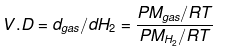V.D = Mgas / MH2
V.D = M/2
V.D = Molecular wt of gas/Molecular wt of H2 gas
The density of Cl2 gas with respect to O2 gas = Molecular wt. of Cl2 gas/Molecular wt. of O2 gas

Q.1. A crystalline hydrated salt, on being rendered anhydrous, loses 45.6% of its mass. The percentage composition of the anhydrous salt is: Al = 10.5%, K = 15.1%, S =24.8% and oxygen = 49.6%. Find the empirical formula of the anhydrous and crystalline hydrated salt. [K = 39; Al = 27; S = 32; O = 16; H = 1]
Ans. The empirical formula of anhydrous salt = KAlS2O8
Hydrated salt composition: % anhydrous part = 54.4% and % H2O = 45.6%
The empirical formula of hydrated salt = KAIS2O8.12H2O

Q.2. A colorless crystalline compound has the following percentage composition: Sulphur 24.24%, nitrogen 21.21%, hydrogen 6.06% and the rest is oxygen. Determine the empirical formula of the compound. If the molecular mass is 132, what is the molecular formula of the compound? Name the compound if it is found to be sulphite.
Ans. Empirical formula SN2H8O4
Molecular formula = SN2H8O4
Name- Ammonium sulphite: (NH4)2SO3

Q.3. A gaseous hydrocarbon contains 85.7% carbon and 14.3% hydrogen. 1 litre of the hydrocarbon weighs 1.26 g at NTP. Determine the molecular formula of the hydrocarbon.
Ans. C2H4

Offer running on EduRev: Apply code STAYHOME200 to get INR 200 off on our premium plan EduRev Infinity!

## Chemistry Class 11

129 videos|212 docs|183 tests

,

,

,

,

,

,

,

,

,

,

,

,

,

,

,

,

,

,

,

,

,

;